Published: 30 September 2017

# Shooting method for free vibration of FGM Reissner-Mindlin circular plates resting on elastic foundation in thermal environments

Qing-Lu Li1
Wei-Di Luan2
Zuo-Quan Zhu3
1, 2, 3Department of Engineering Mechanics, Lanzhou University of Technology, Lanzhou, Gansu 730050, P. R. China
Corresponding Author:
Qing-Lu Li
Views 155

#### Abstract

This paper presents a free vibration analysis of functionally graded Reissner-Mindlin circular plates with various supported boundaries in thermal environments. A FGM consisting of metal and ceramic was considered in the study. Based on the geometric equation, physical equation and equilibrium equation of thick plate, taking into account the transverse shearing deformation, the free vibration equation of the axisymmetric FGM moderately thick circular plates was derived in terms of the middle surface angles of rotation and lateral displacement. The material properties of the plate were assumed to vary continuously in the thickness direction according a power law. By using shooting method to solve the coupled ordinary differential equations with different boundary conditions, the natural frequencies of FGM thick circular plates were obtained numerically. The effects of material gradient property, thickness ratio and boundary conditions on the natural frequencies were discussed in detail.

## 1. Introduction

Functionally graded materials(FGMs), a novel generation of microscopically composites in which the mechanical properties vary smoothly and continuously from one surface to another, proposed in the early 1980s [1, 2]. Structures made from these materials can effectively reduce thermal stress concentration so that they can be used in the high-temperature environments such as aerospace, optics, nuclear and civil engineering. So, the studies of the mechanical behaviors of FGM structures under the mechanical and thermal loadings have being attracted more and more attentions of scientists and also have become a new research field of solid mechanics.

Comprehensive studies on the vibration responses of FGM plates are available in the literatures, but most of which have been limited to analysis of beams and thin circular plates. For example, Xiang and Yang  carried out the free and forced vibration analysis of a laminated FGM Timoshenko beam with variable thickness under heat conduction. Li and Fan  proposed natural frequencies solutions for the free vibration of a FGM beam with a through-width delamination based on the Timoshenko beam theory. Leissa and Narita  investigated natural frequencies of a simply supported circular plate using Classical plate theory with ordinary and modified Bessel functions of the first kind. Kermani at al.  studied the free vibration analysis of multi-directional FGM circular and annular plates. Dong studied  three-dimensional free vibration of FGM annular plates based on Chebyshev-Ritz method. Wang at al.  investigated the axisymmetric free vibration of FGM thin circular plates based on the three-dimensional theory. Malekzadeh at al.  derived governing equations and boundary conditions by Hamilton principle of FGM circular arches with temperature-dependent properties in thermal environment and then gave the in-plane free vibration solutions. Iman at al.  provided an analytic solution for the free vibration of multi-directional FGM circular and annular plates. They used state-space differential quadrature method to find the semi-analytical or numerical solutions based on the three-dimensional theory of elasticity. Lee at al.  provided a semi-analytical solution for the free vibration analysis of circular plate with multiple holes by using the indirect boundary integral method. Ramesh and Mohan Rao  presented the natural frequencies of vibration of a rotating pre-twisted functionally graded cantilever beam. Ying et al.  presented solutions for bending and free vibration of FGM beams resting on a Winkler-Pasternak elastic foundation. By using classical beam theory, Aydogdu and Taskin  investigated the free vibration behavior of a simply supported FGM beam. Pradhan and Chakraverty  carried out the free vibration analysis of FGM thin elliptic plates with various edge supports.

Compared with the free vibration analysis of FGM beams and thin circular plates, the free vibration analysis of FGM thick plates is rare. Chen at al.  investigated the thermal behavior of a thick transversely isotropic FGM rectangular plate based on the three-dimensional elasticity theory. Kim and Lee  developed the geometrically nonlinear isogeometric analysis of FGM plates based on physical neutral surface and first-order shear deformation theory. Foroughi Hamid and Azhari Mojtaba  analyzed the mechanical buckling and free vibration of thick FGM plates resting on elastic foundation using the higher order B-spline finite strip method. Jha at al.  presented deformation and stress analyses of functionally graded thick plates based on the two-dimensional theory. Liu and Lee  provided a vibrations analysis of thick circular and annular plates based on three-dimensional theory with finite element method.

To the author’s best knowledge, in the previous works only the mechanical vibrations of FGM circular plates are investigated and there have been few researches dealing with the free vibration behavior of moderately thick circular plates, especially the effects of thermal environment are not considered. The few researches are Malekzadeh , who studied the free vibration characteristic of tapered Mindlin plates using DQM. Malekzadeh et al.  presented the vibration analysis of FGM thick annular plates subjected to thermal environment based on 3D elasticity theory. Considering the thermal environment effects and using Hamilton's principle, differential quadrature method is adopted to solve the equations of motion and the frequency parameters are obtained. Furthermore, Malekzadeh et al.  extended their work to the free vibration of FGM elastically supported FGM annular plates in thermal environment. Shen and Wang  reported a free vibration analysis of FGM rectangular plates resting on elastic foundations in thermal environments based on Voigt model and Mori-Tanaka model respectively.

Shooting method is found to be a simple and efficient numerical technique for solving non-linear governing equations. Some works have successfully used shooting method to analyze post-buckling, bending and vibration analysis. For example, Li and Zhou  carried out the non-linear vibration and thermal buckling of heated orthotropic circular plates by shooting method. Ma and Lee  investigated the nonlinear mechanical behaviors in-plane thermal loading of a beam made of FGM. Sun and Li  presented the thermal post-buckling of FGM circular plates subjected to transverse poing-space constraints, but they cannot consider the effect of thermal loading.

Therefore, in the present study, free vibration of a FGM moderately thick circular plate in thermal environment is presented by employing the numerical shooting technique. The material properties are thus assumed temperature-dependent and graded in the thickness direction. The equations of motion are derived using the Hamilton’s principle based on the first order shear deformation theory and three different boundary conditions are considered. After that, we employ the shooting method to solve the equations of motion with the related boundary conditions. The effects of uniform and non-uniform temperature rise parameters, gradients of materials properties, thickness ratio and boundary conditions on the natural frequencies are analyzed in detail.

## 2. Problem formulation

Consider a FGM moderately thick circular plate on Winkler foundation with radius $R$ and uniform thickness $h$, which is made from a mixture of ceramic and metals. A cylindrical coordinate system $\left(r,\theta ,z\right)$ with its origin at the center of the mid-plane of the plate is defined, where $r$, $\theta$ and $z$ represent coordinates in the radial, circumferential and thickness directions, respectively. The coordinate system is illustrated in Fig. 1.

Fig. 1Geometry and coordinates of an FGM circular plate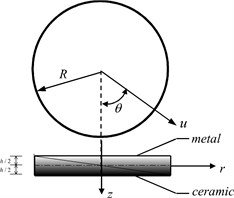### 2.1. Temperature field

Since functionally graded materials are most commonly used in high temperature environment, it is essential to take into consideration this temperature-dependency. Thus, the effective material properties $P$ of the metal and ceramic, such as elasticity modulus, $E\text{,}$ thermal expansion coefficient, $\alpha$, thermal conductivity, $K$, Poisson’s ratio, $\nu$, may be expressed as a nonlinear function of temperature:

1
$P={P}_{0}\left({P}_{-1}{T}^{-1}+1+{P}_{1}T+{P}_{2}{T}^{2}+{P}_{3}{T}^{3}\right).$

In which $T={T}_{0}+\mathrm{\Delta }T$ and ${T}_{0}=$ 300 K (room temperature), the temperature-dependence coefficients ${P}_{-1}$, ${P}_{0}$, ${P}_{1}$, ${P}_{2}$ and ${P}_{3}$ are unique to the constituents.

In this section, we assume that the temperature variation is uniform or occurs in the thickness only. Therefore, the temperature distribution along the thickness direction can be obtained by solving a steady-state one-dimensional heat transfer equation:

2
$-\frac{d}{dz}\left[K\left(z\right)\frac{dT}{dz}\right]=0.$

This equation is solved by imposing boundary condition of $T\left(-\frac{h}{2}\right)={T}_{m}$, $T\left(\frac{h}{2}\right)={T}_{c}$:

3
$T\left(z\right)={T}_{m}+\frac{\mathrm{\Delta }T}{C}{\sum _{i=0}^{\infty }\left(-1{\right)}^{i}\frac{\left({K}_{c}-{K}_{m}{\right)}^{i}}{\left(i×p+1\right){{K}_{m}}^{i}}\left(\frac{1}{2}+\frac{z}{h}\right)}^{i×p+1}.$

In which $\mathrm{\Delta }T={T}_{c}-{T}_{m}$, $C=\sum _{i=0}^{\infty }\left({K}_{c}-{K}_{m}{\right)}^{i}/\left(i×p+1\right){{K}_{m}}^{i}$, where ${K}_{c}$ and ${K}_{m}$ denotes the ceramic and metal thermal conductivity.

### 2.2. Effective material properties of FGMs

According to Voigt rule, the effective material properties $P$ of FGMs may be written as:

4
$P={P}_{c}{V}_{c}+{P}_{m}{V}_{m},$

where the subscripts $c$ and $m$ denote the ceramic and metallic constituents, respectively, ${V}_{c}$ and ${V}_{m}$ denotes the ceramic and metal volume fractions of and they can be expressed by ${V}_{c}+{V}_{m}=1$, the volume fraction ${V}_{c}$ defined a simple power low by:

5
${V}_{c}={\left(\frac{1}{2}+\frac{z}{h}\right)}^{p},$

where $p$ is the gradient index of FGM. Fig. 2 shows the variations of volume fraction of ceramic phase through the thickness of plate for various values of $p$ calculated from Eq. (5).

Fig. 2Variations of the volume fraction of ceramic phase versus the dimensionless thickness of the FGM circular plate for different values of p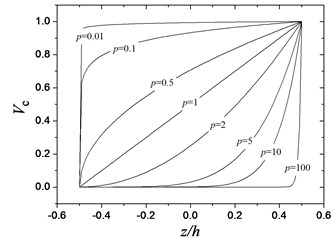For simplicity, the Poisson’s ratio $v$ is assumed to be a constant for functionally graded materials. The effective material properties follow the distribution law of Eqs. (4) and (5), namely:

6a
$E\left(z,T\right)={E}_{c}\left(T\right){\left(\frac{1}{2}+\frac{z}{h}\right)}^{p}+{E}_{m}\left(T\right)\left[1-{\left(\frac{1}{2}+\frac{z}{h}\right)}^{p}\right],$
6b
$\alpha \left(z,T\right)={\alpha }_{c}\left(T\right){\left(\frac{1}{2}+\frac{z}{h}\right)}^{p}+{\alpha }_{m}\left(T\right)\left[1-{\left(\frac{1}{2}+\frac{z}{h}\right)}^{p}\right],$
6c
$K\left(z,T\right)={K}_{c}\left(T\right){\left(\frac{1}{2}+\frac{z}{h}\right)}^{p}+{K}_{m}\left(T\right)\left[1-{\left(\frac{1}{2}+\frac{z}{h}\right)}^{p}\right],$
6d
$\nu \left(z\right)=\nu ,$
6e
$\rho \left(z\right)={\rho }_{c}{\left(\frac{1}{2}+\frac{z}{h}\right)}^{p}+{\rho }_{m}\left[1-{\left(\frac{1}{2}+\frac{z}{h}\right)}^{p}\right],$

### 2.3. Governing equations

According to one-order shear plate theory, it can be assumed that the axisymmetric displacement field are:

7a
${U}_{r}\left(r,z,t\right)=z\psi \left(r,t\right),$
7b
${U}_{\theta }\left(r,z,t\right)=0,$
7c
${U}_{z}\left(r,z,t\right)=w\left(r,t\right).$

In which ${U}_{r}$, ${U}_{\theta }$ and ${U}_{z}$ are total displacement components along the coordinates $r$, $\theta$ and $z$, respectively, $u$ and $w$ are the displacements in the mid-plane of the plate along the coordinates $r$ and $z$, respectively, and $\psi$ denotes the slope at $z=$ 0 of the deformed line that was straight in the undeformed plate, $t$ is the time variable.

The strains can be expressed as follow:

8a
${\epsilon }_{r}=\frac{\partial {U}_{r}}{\partial r},$
8b
${\epsilon }_{\theta }=\frac{{U}_{r}}{r},$
8c
${\gamma }_{rz}=\frac{\partial {U}_{r}}{\partial z}+\frac{\partial {U}_{z}}{\partial \theta }.$

Considering nonlinear strain-displacement relationships, one then obtains:

9a
${\epsilon }_{r}=\frac{1}{2}{\left(\frac{\partial w}{\partial r}\right)}^{2}+z\frac{\partial \psi }{\partial r},$
9b
${\epsilon }_{\theta }=z\frac{\psi }{r},$
9c
${\gamma }_{rz}=\psi +\frac{\partial w}{\partial r},$

where ${\epsilon }_{r}$ and ${\epsilon }_{\theta }$ are the normal strains and ${\gamma }_{rz}$ is the shear strain.

Based on Hooke’s law, the stress-strain relations are expressed by following formulations:

10
${\sigma }_{r}=\frac{E\left(z,T\right)}{1-{\nu }^{2}}\left({\epsilon }_{r}+\nu {\epsilon }_{\theta }\right)-\frac{E\left(z,T\right)\alpha \left(z,T\right)}{1-\nu }T\left(z\right),$
11
${\sigma }_{\theta }=\frac{E\left(z,T\right)}{1-{\nu }^{2}}\left({\epsilon }_{\theta }+\nu {\epsilon }_{r}\right)-\frac{E\left(z,T\right)\alpha \left(z,T\right)}{1-\nu }T\left(z\right),$
12
${\tau }_{rz}=\frac{E\left(z,T\right)}{2\left(1+\nu \right)}{\gamma }_{rz}.$

The stress can then be determined in terms of the mid-plane displacement through Eqs. (9a)-(9c), they can be calculated as:

13
${\sigma }_{r}=\frac{E\left(z,T\right)}{1-{\nu }^{2}}\left[\frac{1}{2}{\left(\frac{\partial w}{\partial r}\right)}^{2}+z\left(\frac{\partial \psi }{\partial r}+\nu \frac{\psi }{r}\right)\right]-\frac{E\left(z,T\right)\alpha \left(z,T\right)}{1-\nu }T\left(z\right),$
14
${\sigma }_{\theta }=\frac{E\left(z,T\right)}{1-{\nu }^{2}}\left[\frac{\nu }{2}{\left(\frac{\partial w}{\partial r}\right)}^{2}+z\left(\frac{\psi }{r}+\nu \frac{\partial \psi }{\partial r}\right)\right]-\frac{E\left(z,T\right)\alpha \left(z,T\right)}{1-\nu }T\left(z\right),$
15
${\tau }_{rz}=\frac{E\left(z,T\right)}{2\left(1+\nu \right)}\left(\psi +\frac{\partial w}{\partial r}\right).$

The membrane forces, the bending moments and the shear resultant force can be deduced from thickness integration of Eqs. (13), (14) and Eq. (15) respectively:

16a
${N}_{r}=\underset{-0.5h}{\overset{0.5h}{\int }}{\sigma }_{r}dz={A}_{11}\frac{1}{2}{\left(\frac{\partial w}{\partial r}\right)}^{2}+{B}_{11}\left(\frac{\partial \psi }{\partial r}+\nu \frac{\psi }{r}\right)-{N}_{T},$
16b
${N}_{\theta }=\underset{-0.5h}{\overset{0.5h}{\int }}{\sigma }_{\theta }dz={A}_{11}\frac{\nu }{2}{\left(\frac{\partial w}{\partial r}\right)}^{2}+{B}_{11}\left(\frac{\psi }{r}+\nu \frac{\partial \psi }{\partial r}\right)-{N}_{T},$
16c
${M}_{r}=\underset{-0.5h}{\overset{0.5h}{\int }}{\sigma }_{r}zdz={B}_{11}\frac{1}{2}{\left(\frac{\partial w}{\partial r}\right)}^{2}+{D}_{11}\left(\frac{\partial \psi }{\partial r}+\nu \frac{\psi }{r}\right)-{M}_{T},$
16d
${M}_{\theta }=\underset{-0.5h}{\overset{0.5h}{\int }}{\sigma }_{\theta }zdz={B}_{11}\frac{\nu }{2}{\left(\frac{\partial w}{\partial r}\right)}^{2}+{D}_{11}\left(\frac{\psi }{r}+\nu \frac{\partial \psi }{\partial r}\right)-{M}_{T},$
16e
${Q}_{r}=\underset{-0.5h}{\overset{0.5h}{\int }}{\tau }_{rz}dz=\frac{S}{{\kappa }_{s}}\left(\psi +\frac{\partial w}{\partial r}\right).$

Here ${\kappa }_{s}$ is the shear correction factor, for Mindlin plate ${\kappa }_{s}=12/{\pi }^{2}$, Reissner plate ${\kappa }_{s}=$ 6/5. ${A}_{11}$, ${B}_{11}$, ${D}_{11}$ and $S$ are the stretching, bending-stretching coupling, bending and shear rigidity coefficient respectively; and the thermal membrane force ${N}_{T}$ and thermal bending moment ${M}_{T}$ can be calculated by following expressions:

17
$\left({A}_{11},{B}_{11},{D}_{11}\right)=\underset{-0.5h}{\overset{0.5h}{\int }}\frac{E\left(z,T\right)}{1-{\nu }^{2}}\left(1,z,{z}^{2}\right)dz,S={\int }_{-h/2}^{h/2}\frac{E\left(z\right)}{2\left(1+\nu \right)}dz,$
18
$\left({N}_{T},{M}_{T}\right)=\underset{-0.5h}{\overset{0.5h}{\int }}\frac{E\left(z,T\right)\alpha \left(z,T\right)}{1-\nu }T\left(z\right)\left(1,z\right)dz.$

Substitution of Eq. (6a) and Eq. (3) into Eq. (17) and Eq. (18) gives following statements:

$\left({A}_{11},{B}_{11},{D}_{11}\right)=\frac{{E}_{c}\left(T\right)}{1-{\nu }^{2}}\left(h{f}_{1},{h}^{2}{f}_{2},{h}^{3}{f}_{3}\right),S=\frac{{E}_{c}\left(T\right)}{2\left(1+\nu \right)}h{f}_{1},$
$\left({N}_{T},{M}_{T}\right)=\frac{{E}_{c}\left(T\right){\alpha }_{c}\left(T\right)}{1-\nu }\underset{-0.5h}{\overset{0.5h}{\int }}\left({T}_{m}{f}_{4}+\frac{\mathrm{\Delta }T}{C}{f}_{5}\right)\left(1,z\right)dz.$

Note that${E}_{r}={E}_{m}/{E}_{c}\text{,}$${\alpha }_{r}={\alpha }_{m}/{\alpha }_{c}\text{,}$${K}_{r}={K}_{m}/{K}_{c}$, ${f}_{i}\left(i=1,2,3,4,5\right)$ are coefficients determined as follows:

${f}_{1}={E}_{r}+\frac{1-{E}_{r}}{p+1},$
${f}_{2}=\frac{p\left(1-{E}_{r}\right)}{2\left(p+1\right)\left(p+2\right)},$
${f}_{3}=\frac{{E}_{r}}{12}+\frac{\left({p}^{2}+p+2\right)\left(1-{E}_{r}\right)}{4\left(p+1\right)\left(p+2\right)\left(p+3\right)},$
${f}_{4}=\left[{E}_{r}+\left(1-{E}_{r}\right){\left(\frac{1}{2}+\frac{z}{h}\right)}^{p}\right]\left[{\alpha }_{r}+\left(1-{\alpha }_{r}\right){\left(\frac{1}{2}+\frac{z}{h}\right)}^{p}\right],$
${f}_{4}=\left[{E}_{r}+\left(1-{E}_{r}\right){\left(\frac{1}{2}+\frac{z}{h}\right)}^{p}\right]\left[{\alpha }_{r}+\left(1-{\alpha }_{r}\right){\left(\frac{1}{2}+\frac{z}{h}\right)}^{p}\right]\sum _{i=0}^{\infty }{\left(-1\right)}^{i}\frac{{\left(1-{K}_{r}\right)}^{i}}{\left(i×p+1\right){K}_{r}^{i}}{\left(\frac{1}{2}+\frac{z}{h}\right)}^{i×p+1}$

### 2.4. Motion equations

The kinetic energy $T$ and the elastic potential ${V}_{e}$ of the FGM plate may be written as:

19
$T=\frac{1}{2}\underset{\mathrm{\Omega }}{\int }\rho \left[{\left(\frac{\partial {U}_{r}}{\partial t}\right)}^{2}+{\left(\frac{\partial {U}_{z}}{\partial t}\right)}^{2}\right]d\mathrm{\Omega },$
20
${V}_{e}=\frac{1}{2}\underset{\mathrm{\Omega }}{\int }\left({\sigma }_{r}{\epsilon }_{r}+{\sigma }_{\theta }{\epsilon }_{\theta }+{\tau }_{rz}{\gamma }_{rz}\right)d\mathrm{\Omega },$

where $\rho =\rho \left(z,T\right)$ is the mass density of the FGM plate, and $\mathrm{\Omega }$denotes domain of the FGM plate.

The load-displacement relationship of the foundation is assumed to be $q={k}_{w}w-{k}_{g}{\nabla }^{2}w$, where $q$ is the force per unit area, and $\nabla$ is Laplace differential operator; ${k}_{w}$ is the Winkler foundation stiffness and ${k}_{g}$ is a constant showing the effect of the shear interactions of the vertical elements.

The elastic foundation energy having shear deformable layers ${V}_{k}$ of the plate is expressed as follows:

21
${V}_{k}=\frac{1}{2}\underset{A}{\iint }\left[{k}_{w}{w}^{2}-{k}_{g}{\left(\frac{\partial w}{\partial r}\right)}^{2}\right]dA.$

### 2.5. Hamilton’s principle

The free vibration equations of motion will be derived according Hamilton’s principle, which has the following form:

22
$\delta \mathrm{\Pi }=\underset{{t}_{1}}{\overset{{t}_{2}}{\int }}\delta \left(T-{V}_{e}-{V}_{k}\right)dt,$

where ${t}_{1}$ and ${t}_{2}$ are the beginning and end of motion time, respectively, in which the virtual kinetic energy is:

23
$\delta T=\underset{A}{\iint }{I}_{0}\frac{\partial w}{\partial t}\delta \frac{\partial w}{\partial t}dA+\underset{A}{\iint }{I}_{2}\frac{\partial \psi }{\partial t}\delta \frac{\partial \psi }{\partial t}dA.$

The virtual elastic potential and elastic foundation energy may be expressed as follows:

24
$\delta {V}_{e}=\underset{\mathrm{\Omega }}{\int }\left({\sigma }_{r}\delta {\epsilon }_{r}+{\sigma }_{\theta }\delta {\epsilon }_{\theta }+{\tau }_{rz}\delta {\gamma }_{rz}\right)d\mathrm{\Omega }=\underset{A}{\iint }\left({N}_{r}\frac{\partial w}{\partial r}\delta \frac{\partial w}{\partial r}+{M}_{r}\delta \frac{\partial \psi }{\partial r}+\frac{{M}_{\theta }}{r}\delta \psi +{Q}_{r}\delta \psi +{Q}_{r}\delta \frac{\partial w}{\partial r}\right)dA.$
25
$\delta {V}_{k}=\underset{A}{\iint }\left[{k}_{w}w\delta w-{k}_{g}\left(\frac{1}{r}\frac{\partial w}{\partial r}+\frac{{\partial }^{2}w}{\partial {r}^{2}}\right)\delta w\right]dA.$

In which:

$\left({I}_{0},{I}_{2}\right)={\int }_{-\frac{h}{2}}^{\frac{h}{2}}\rho \left(z\right)\left(1,{z}^{2}\right)dz={\rho }_{c}\left(h{f}_{6},{h}^{3}{f}_{7}\right),$

where:

${f}_{6}={\rho }_{r}+\frac{1-{\rho }_{r}}{p+1},{f}_{7}=\frac{{\rho }_{r}}{12}+\frac{\left({p}^{2}+p+2\right)\left(1-{\rho }_{r}\right)}{4\left(p+1\right)\left(p+2\right)\left(p+3\right)},{\rho }_{r}=\frac{{\rho }_{m}}{{\rho }_{c}}.$

Applying integrating by parts and collecting the coefficients of $\delta \psi$, $\delta w$, the governing equations of motion can be expressed as:

26a
$\delta \psi :\frac{\partial {M}_{r}}{\partial r}+\frac{{M}_{r}-{M}_{\theta }}{r}-{Q}_{r}={I}_{2}\frac{{\partial }^{2}\psi }{\partial {t}^{2}},$
26b
$\delta w:\frac{\partial {Q}_{r}}{\partial r}+\frac{{Q}_{r}}{r}+\frac{1}{r}\frac{\partial }{\partial r}\left(r{N}_{r}\frac{\partial w}{\partial r}\right)-{k}_{w}w+{k}_{g}\left(\frac{{\partial }^{2}w}{\partial {r}^{2}}+\frac{1}{r}\frac{\partial w}{\partial r}\right)={I}_{0}\frac{{\partial }^{2}w}{\partial {t}^{2}}.$

Inserting Eqs. (16a)-(16e) into Eqs. (24a, b) and regardless of nonlinear terms, the governing equations of motion can be expressed as:

27a
$D\left(\frac{{\partial }^{2}\psi }{\partial {r}^{2}}+\frac{1}{r}\frac{\partial \psi }{\partial r}-\frac{\psi }{{r}^{2}}\right)-\frac{S}{{\kappa }_{s}}\left(\psi +\frac{\partial w}{\partial r}\right)={I}_{2}\frac{{\partial }^{2}\psi }{\partial {t}^{2}},$
27b
$\frac{S}{{\kappa }_{s}}\left(\frac{\partial \psi }{\partial r}+\frac{{\partial }^{2}w}{\partial {r}^{2}}+\frac{\psi }{r}+\frac{1}{r}\frac{\partial w}{\partial r}\right)-{N}_{T}\left(\frac{{\partial }^{2}w}{\partial {r}^{2}}+\frac{1}{r}\frac{\partial w}{\partial r}\right)-{k}_{w}w+{k}_{g}\left(\frac{{\partial }^{2}w}{\partial {r}^{2}}+\frac{1}{r}\frac{\partial w}{\partial r}\right)={I}_{0}\frac{{\partial }^{2}w}{\partial {t}^{2}}.$

Assume that the vibration of circular plate is harmonic. Then, the dynamic response can be expressed as:

28a
$w\left(r,t\right)=\stackrel{~}{w}\left(r\right)\mathrm{c}\mathrm{o}\mathrm{s}\mathrm{\Omega }t,$
28b
$\psi \left(r,t\right)=\stackrel{~}{\psi }\left(r\right)\mathrm{c}\mathrm{o}\mathrm{s}\mathrm{\Omega }t,$

where $\mathrm{\Omega }$ is the natural frequency, and $\stackrel{~}{w}\left(r\right)$ and $\stackrel{~}{\psi }\left(r\right)$ are the shape functions. Substituting Eqs. (28a) and (28b) into Eqs. (27a) and (27b) gives the governing equations of motion of the problem as follow:

29a
$D\left(\frac{{d}^{2}\stackrel{~}{\psi }}{d{r}^{2}}+\frac{1}{r}\frac{d\stackrel{~}{\psi }}{dr}-\frac{\stackrel{~}{\psi }}{{r}^{2}}\right)-\frac{S}{{\kappa }_{s}}\left(\stackrel{~}{\psi }+\frac{d\stackrel{~}{w}}{dr}\right)=-{I}_{2}\stackrel{~}{\psi }{\mathrm{\Omega }}^{2},$
29b
$\frac{S}{{\kappa }_{s}}\left(\frac{d\stackrel{~}{\psi }}{dr}+\frac{{d}^{2}\stackrel{~}{w}}{d{r}^{2}}+\frac{\stackrel{~}{\psi }}{r}+\frac{1}{r}\frac{d\stackrel{~}{w}}{dr}\right)-{N}_{T}\left(\frac{{d}^{2}\stackrel{~}{w}}{d{r}^{2}}+\frac{1}{r}\frac{d\stackrel{~}{w}}{dr}\right)-{k}_{w}\stackrel{~}{w}+{k}_{g}\left(\frac{{d}^{2}\stackrel{~}{w}}{d{r}^{2}}+\frac{1}{r}\frac{d\stackrel{~}{w}}{dr}\right)=-{I}_{0}\stackrel{~}{w}{\mathrm{\Omega }}^{2}.$

Non-dimensional variables are introduced as follows:

$\xi =\frac{r}{R},W=\frac{\stackrel{~}{w}}{R},\delta =\frac{h}{R},Kw=\frac{12\left(1-{\nu }^{2}\right){k}_{w}{R}^{4}}{{E}_{c}{h}^{3}},Kg=\frac{12\left(1-{\nu }^{2}\right){k}_{g}{R}^{2}}{{E}_{c}{h}^{3}},$
${\omega }_{n}=\mathrm{\Omega }{R}^{2}\sqrt{\frac{12\left(1-{\nu }^{2}\right){\rho }_{c}h}{{E}_{c}{h}^{3}}}.$

Substitution of the above non-dimensional transformation into Eqs. (29a) and (29b) gives the governing equations of the FGM Reissner-Mindlin circular plates in dimensionless forms as:

30
$12{\delta }^{2}{f}_{3}\left(\frac{{d}^{2}\stackrel{~}{\psi }}{d{\xi }^{2}}+\frac{1}{\xi }\frac{d\stackrel{~}{\psi }}{d\xi }-\frac{\stackrel{~}{\psi }}{{\xi }^{2}}\right)-\frac{6\left(1-\nu \right)}{{\kappa }_{s}}{f}_{1}\left(\stackrel{~}{\psi }+\frac{dW}{d\xi }\right)=-{\delta }^{4}{f}_{7}\stackrel{~}{\psi }{\omega }_{n}^{2},$
31
$\frac{6\left(1-\nu \right)}{\kappa }{f}_{1}\left(\frac{{d}^{2}W}{d{\xi }^{2}}+\frac{1}{\xi }\frac{dW}{d\xi }+\frac{d\stackrel{~}{\psi }}{d\xi }+\frac{\stackrel{~}{\psi }}{\xi }\right)-{\delta }^{2}{K}_{w}W+\left({\delta }^{2}{K}_{g}-{{N}^{\text{'}}}_{T}\right)\left(\frac{{d}^{2}W}{d{\xi }^{2}}+\frac{1}{\xi }\frac{dW}{d\xi }\right)=-{\delta }^{2}{f}_{6}W{\omega }_{n}^{2}.$

In which:

${N\text{'}}_{T}=12\left(1+\nu \right){\alpha }_{c}\left({T}_{m}\underset{0}{\overset{1}{\int }}{g}_{1}dt+\frac{\mathrm{\Delta }T}{C}\underset{0}{\overset{1}{\int }}{g}_{2}dt\right),$

where:

${g}_{1}=\left[{E}_{r}+\left(1-{E}_{r}\right){t}^{p}\right]\left[{\alpha }_{r}+\left(1-{\alpha }_{r}\right){t}^{p}\right],$
${g}_{2}=\left[{E}_{r}+\left(1-{E}_{r}\right){t}^{p}\right]\left[{\alpha }_{r}+\left(1-{\alpha }_{r}\right){t}^{p}\right]\sum _{i=0}^{\infty }\left(-1{\right)}^{i}\frac{\left(1-{K}_{r}{\right)}^{i}}{\left(i×p+1\right){{K}_{r}}^{i}}{t}^{i×p+1}.$

In the present study, three traditional boundary conditions are considered. Boundary conditions in dimensions form can be written as:

32
$\stackrel{~}{\psi }=0,\frac{dW}{d\xi }+\stackrel{~}{\psi }=0,\xi =0.$

Clamped edge (C):

33
$\stackrel{~}{\psi }=0,W=0,\xi =1.$

Simply supported edge (S):

34
$\frac{d\stackrel{~}{\psi }}{d\xi }+\nu \stackrel{~}{\psi }=0,W=0,\xi =1.$

Free edge (F):

35
$\frac{d\stackrel{~}{\psi }}{d\xi }+\nu \stackrel{~}{\psi }=0,\frac{dW}{d\xi }+\stackrel{~}{\psi }=0,\xi =1.$

## 3. Shooting method

Due to complexity and the coupling of these partial differential equations, it is very different to obtain any analytical solution of this problem. In what follows, a shooting method [25-27] is employed to numerically solve the problems. Here, the governing Eqs. (30) and (31) and boundary conditions Eqs. (32)-(33), (32)-(34) and (32)-(35) can be rewritten in the standard form:

36a
$\frac{d\mathbf{Y}}{d\xi }=\mathbf{H}\left(\xi ,Y\right),\left(0<\xi <1\right),$
36b
${\mathbf{B}}_{0}Y\left(0\right)={b}_{0},$
36c
${\mathbf{B}}_{1}Y\left(1\right)={b}_{1},$

where:

$\mathbf{Y}={\left[{y}_{1},{y}_{2},{y}_{3},{y}_{4},{y}_{5}\right]}^{T}={\left[\stackrel{~}{\psi },\frac{d\stackrel{~}{\psi }}{d\xi },W,\frac{dW}{d\xi },{\omega }_{n}\right]}^{T},$
$\mathbf{H}={\left[{y}_{2},{\mathrm{\Delta }}_{1},{y}_{4},{\mathrm{\Delta }}_{2},0\right]}^{T}.$

Expressions of ${\mathrm{\Delta }}_{1}$, ${\mathrm{\Delta }}_{2}$ and ${b}_{1}$, ${b}_{2}$ are as follows:

${\mathrm{\Delta }}_{1}=\frac{{y}_{1}}{{\xi }^{2}}-\frac{{y}_{2}}{\xi }+\frac{\left(1-\nu \right)}{2{\delta }^{2}{\kappa }_{s}}\frac{{f}_{1}}{{f}_{{}_{3}}}\left({y}_{1}+{y}_{4}\right)-\frac{{\delta }^{2}}{12}\frac{{f}_{7}}{{f}_{3}}{y}_{1}{{y}_{5}}^{2},$
${\mathrm{\Delta }}_{2}=\frac{\left[-\frac{6\left(1-\nu \right)}{\kappa }{f}_{1}\left(\frac{{y}_{4}}{\xi }+\frac{{y}_{1}}{\xi }+{y}_{2}\right)+\left({N\text{'}}_{T}-{\delta }^{2}{K}_{g}\right)\frac{{y}_{4}}{\xi }+{\delta }^{2}{K}_{w}{y}_{3}-{\delta }^{2}{f}_{6}{y}_{3}{{y}_{5}}^{2}\right]}{\left[\frac{6\left(1-\nu \right)}{\kappa }{f}_{1}+\left({\delta }^{2}{K}_{g}-{N}_{T}^{\text{'}}\right)\right]},$
${b}_{0}={\left\{\begin{array}{lll}0& \eta & 0\end{array}\right\}}^{T},{b}_{1}={\left\{\begin{array}{ll}0& 0\end{array}\right\}}^{T},$

where $\eta$ is a dimensionless deflection parameter.

${\mathbf{B}}_{0}$ and ${\mathbf{B}}_{1}$ are matrixes of order 3×5 and 2×5 respectively, they are:

${\mathbf{B}}_{0}=\left[\begin{array}{lllll}1& 0& 0& 0& 0\\ 0& 0& 1& 0& 0\\ 0& 0& 0& 1& 0\end{array}\right].$

For the clamped boundary:

${\mathbf{B}}_{1}=\left[\begin{array}{lllll}1& 0& 0& 0& 0\\ 0& 0& 1& 0& 0\end{array}\right].$

For the Simply supported boundary:

${\mathbf{B}}_{1}=\left[\begin{array}{lllll}\nu & 1& 0& 0& 0\\ 0& 0& 1& 0& 0\end{array}\right].$

For the free boundary:

${\mathbf{B}}_{1}=\left[\begin{array}{lllll}1& 0& 0& 0& 0\\ 0& 0& 0& 1& 0\end{array}\right].$

Consider the initial value problem:

37a
$\frac{dG}{d\xi }=\mathbf{H}\left(\xi ,G\right),$
37b
$G\left(0\right)=\mathbf{I}\left(\eta ,\mathbf{D}\right),$

where $G={\left\{{g}_{1},{g}_{2},{g}_{3},{g}_{4},{g}_{5}\right\}}^{T}$, $I={\left\{0,{d}_{1},\eta ,0,{d}_{2}\right\}}^{T}$, and $D={\left\{{d}_{1},{d}_{2}\right\}}^{T}$ is the initial parameter vector.

The unique solution for the initial value problem must exist, namely:

38
$\mathbf{G}\left(\xi ;\eta ,\mathbf{D}\right)=I\left(\eta ,\mathbf{D}\right)+\underset{0}{\overset{\xi }{\int }}H\left(\zeta ,\mathbf{G}\right)d\zeta .$

For given value of $\eta$, we seek components of ${D}^{*}={\left\{{d}_{1}^{*},{d}_{2}^{*}\right\}}^{T}$ such that a solution of Eq. (38) satisfies boundary condition Eq. (37b), that is:

39
${\mathbf{B}}_{1}\mathbf{G}\left(\xi ;\eta ,{\mathbf{D}}^{*}\right)={\left\{0，0\right\}}^{T}.$

Obviously, if $\mathbf{D}={\mathbf{D}}^{*}$ is a root of Eq. (39), the solution of the boundary-value problem Eq. (36) is then obtained as:

40
$\mathbf{Y}\left(\xi \right)=\mathbf{G}\left(\xi ;\eta ,{\mathbf{D}}^{*}\right).$

We employ the Runge-Kutta method to integrate the system Eq. (38) of ordinary differential equations, and the Newton-Raphson iteration method to search for a root ${\mathbf{D}}^{*}$ of Eq. (39) to find a numerical solution of the boundary-value problem Eq. (36). This approach is called a shooting method.

## 4. Shooting method for approximate solutions of free vibration of FGM Reissner-Mindlin plates

In what follows, the material properties of ceramic, $Zr{O}_{2}$, and metallic, Ti-6Al-4V, as given in Table 1, are used in the numerical computations.

### 4.1. Comparison studies

To ensure the accuracy and effectiveness of shooting method, two examples are solved for free vibration of homogeneous and FGM Mindlin circular plate.

Table 1Temperature-dependent coefficients for ceramic and metals from Reddy and Chin 

 Material Proprieties ${P}_{-1}$ ${P}_{0}$ ${P}_{1}$ ${P}_{2}$ ${P}_{3}$ Ti-6Al-4V $E$ (GPa) 0 122.7e+9 –4.605e-4 –4.605-4 0 $Zr{O}_{2}$ $\alpha$ (1/K) 0 7.5788e-6 6.638e-4 –3.147e-6 0 $\kappa$ (W/mK) 0 1.0 1.704e-2 0 0 $\rho$ (kg·m-3) 0 4229 0 0 0 $E$ (GPa) 0 244.27e+9 –1.371e-3 1.214e-6 –3.681e-10 $\alpha$ (1/K) 0 12.766e-6 –1.491e-3 1.006e-5 0 $\kappa$ (W/m K) 0 1.7 1.276e-4 6.648e-8 0 $\rho$ (kg·m-3) 0 3000 0 0 0

Example 1. When the power law index $p$ equals zero, the functionally graded material is reduced to the homogeneous ceramics Mindlin circular plate. In order to show the accuracy of the present numerical method, by giving $p=$ 0, $\nu =$ 0.3, ${K}_{w}={K}_{g}=$ 0, $\delta =$ 0.2，the comprehensive comparison between the shooting method results and those in Ref.  is given in Table 2, which includes the cases of above-mentioned three sets of boundary conditions. Obviously, the first four lower-order dimensionless natural frequencies show good agreement with the existing results.

Example 2. The first three order dimensionless fundamental frequency parameters $\omega$ of the FGM thick circular plates for the three traditional boundary conditions are calculated and compared in Table 3 with a set of results  based on Rayleigh-Ritz method. The present results are in good agreement with existing results.

Tables 2-3 show that the present results agree well existing results, and thus the accuracy of the shooting method technique are confirmed.

Table 2The first fourth dimensionless natural frequencies ωn for thick circular plates under three sets of boundary conditions

 Boundary condition ${\omega }_{1}$ ${\omega }_{2}$ ${\omega }_{3}$ ${\omega }_{4}$ $C$ Present 9.2401 30.211 56.683 85.572  9.2400 30.211 56.682 85.571 $S$ Present 4.7773 24.995 52.514 82.767  4.7777 24.994 52.514 82.766 $F$ Present – 8.5051 31.111 59.646  – 8.5050 31.111 59.645

Table 3Dimensionless natural frequencies ωn of FGM Mindlin plates with different graded index δ= 0.01

 Boundary condition ${\omega }_{n}$ $p=$ 0.0 $p=$ 0.1 $p=$ 0.5 $p=$ 1 $p=$ 2 $p=$ 5 $C$ 1 Present 10.213 9.9836 9.4503 9.1331 8.815 8.591  10.216 9.8510 8.973 8.500 8.125 7.576 2 Present 39.734 38.843 36.767 35.532 33.423 33.423  39.773 38.352 34.933 33.093 29.496 29.496 $S$ 1 Present 4.935 4.823 4.5673 4.4133 4.2684 4.1426  4.935 4.759 4.335 4.106 3.925 3.659 2 Present 29.704 29.036 27.486 26.564 25.416 24.667  29.736 28.674 26.118 24.742 23.651 22.052 $F$ 2 Present 8.9686 8.7673 8.2986 8.0204 7.7737 5.9883  9.003 8.682 7.908 7.491 7.161 6.677 3 Present 37.787 36.939 34.964 33.793 32.754 25.2045  37.564 36.221 32.993 31.225 29.877 27.857

### 4.2. Numerical results and discussions

In this section, the free vibration of a FGM thick circular plate is numerically analyzed in thermal environment. Table 4 shows the first three order dimensionless fundamental frequency parameters $\omega$ of the FGM thick circular plates for clamped boundary condition changing with the power law index $p$ in room temperature fields without elastic foundation. It can be seen that all the frequencies decrease with the increasing of power law index $p$, which is due to the fact that the decrease of rigidity of the plate, or the increase of the component of metallic in the plate. Also, along with the increase in the value of $\delta$, the frequencies decrease.

Table 4Dimensionless natural frequencies ωn of FGM thick circular plates with clamped edge (ν= 0.3, Tc=Tm= 300 K, Kw=Kg= 0)

 $\delta$ $\omega$ Mindlin solution Reissner solution $p=$ 0.0 $p=$ 0.2 $p=$ 0.5 $p=$ 1 $p=$ 2 $p=$ 5 $p=$ 0 $p=$ 0.2 $p=$ 0.5 $p=$ 1 $p=$ 2 $p=$ 5 0.2 1 8.6948 7.9758 7.3639 6.8684 6.4509 6.0217 8.7051 7.9850 7.3724 6.8763 6.4587 6.0291 2 29.464 27.028 24.908 23.111 21.529 19.931 29.538 27.095 24.969 23.168 21.585 19.983 3 55.752 51.185 47.158 43.659 40.514 37.355 55.944 51.358 47.317 43.808 40.655 37.488 0.3 1 8.1034 7.3952 6.8503 6.3628 5.9388 5.5085 8.1201 7.4468 6.8642 6.3759 5.9514 5.5206 2 24.245 22.211 20.516 18.984 17.599 16.213 24.333 22.347 20.589 19.053 17.665 16.274 3 43.037 39.484 36.446 33.674 31.129 28.594 43.226 39.731 36.604 33.822 31.267 28.723

Subjected to room temperature or heat conduction, the fundamental frequencies decrease significantly with increasing the value of $\delta$ in Fig. 3 and 4. It can be found that for a giving $\delta$, Ceramic plate provide the largest fundamental frequency while the metal plate hold the smallest one. Table 5 shows the effect of uniform temperature rise on the first three-order dimensionless frequency parameters for FGM Mindlin plate with $\delta =$ 0.2 and clamped ends that frequencies monotonously decrease as uniform temperature rise increase.

Fig. 3Fundamental dimensionless frequency parameter ω1 of clamped plate versus δ in room temperature field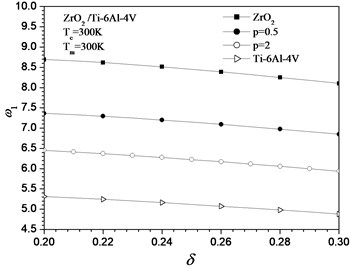Fig. 4Fundamental dimensionless frequency parameter ω1 of clamped plate versus δ subjected to heat conduction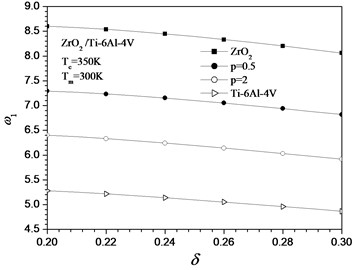Table 5Dimensionless natural frequencies ωn of FGM thick circular plates with clamped edge (ν= 0.3, δ= 0.2, Kw=Kg= 0)

 $\delta$ $\omega$ ${T}_{c}={T}_{m}=$ 400 K ${T}_{c}={T}_{m}=$ 500 K $p=$ 0.0 $p=$ 0.2 $p=$ 0.5 $p=$ 1 $p=$ 2 $p=$ 5 $p=$ 0 $p=$ 0.2 $p=$ 0.5 $p=$ 1 $p=$ 2 $p=$ 5 1 8.5046 7.8108 7.2219 6.7486 6.3529 5.9435 8.3095 7.6418 7.0768 6.6245 6.2531 5.8641 2 29.211 26.808 24.719 22.950 21.397 19.823 28.956 26.588 24.528 22.788 21.263 19.715 3 55.439 50.914 46.924 43.460 40.347 37.219 55.124 50.642 46.689 43.259 40.180 37.084

In order to compare uniform and non-uniform temperature rise effects, average temperature of non-uniform parameters equal to the temperature of uniform case are considered. Table 6 shows the effect of uniform temperature rise and heat conduction on the free vibration of FGM Mindlin circular plates without elastic foundation. It can be observed that for the same value of both uniform and non-uniform rise, the first-three frequency parameters of FGM Mindlin plate decrease with increasing the power law index $p$. One can see that the frequency in case of uniform heating is smaller than that of the non-uniform temperature rise.

Table 6Fundamental dimensionless frequencies ω1 of FGM thick circular plates with clamped edge subject to temperature rise and heat conduction (ν= 0.3, δ= 0.2, Kw=Kg= 0)

 $\omega$ $p=$ 0 $p=$ 0.1 $p=$ 0.5 $p=$ 1 $p=$ 2 $p=$ 5 $p=$ 100 ${T}_{c}={T}_{m}=$ 325 K 8.6484 8.4774 8.0844 7.8528 7.6488 7.4601 7.2487 ${T}_{c}={T}_{m}=$ 350 K 8.6008 8.4334 8.0468 7.8198 7.6210 7.4355 7.2278 ${T}_{c}={T}_{m}=$ 375 K 8.5528 8.3881 8.0091 7.7875 7.5922 7.4117 7.2069 ${T}_{c}=$ 350 K, ${T}_{m}=$ 300 K 8.6873 8.5146 8.1137 7.8788 7.6715 7.4789 7.2664 ${T}_{c}=$ 400 K, ${T}_{m}=$ 300 K 8.6797 8.5063 8.1067 7.8719 7.6648 7.4740 7.2632 ${T}_{c}=$ 450 K, ${T}_{m}=$ 300 K 8.6437 8.4988 8.0996 7.8650 7.6589 7.4691 7.2592

The effects of two-parameter elastic foundation on the first-three frequency parameters of the FGM thick circular plates in thermal environment are exhibited in Table 7. As we expect, the elastic foundation has significant effects on the first-three frequencies of FGM Mindlin plates subjected to uniform temperature and heat conduction, the results are bigger than the frequencies without elastic foundation. Obviously, additional elastic foundation leads frequency rise due to the decrease of the flexibility and the deformation capacity.

Table 7Dimensionless natural frequencies ωn of FGM thick circular plates with clamped edge (ν= 0.3, Kw= 50, Kg= 5)

 $\delta$ $\omega$ ${T}_{c}={T}_{m}=$ 325 K ${T}_{c}=$ 350 K, ${T}_{m}=$ 300 K $p=$ 0 $p=$ 0.2 $p=$ 0.5 $p=$ 1 $p=$ 2 $p=$ 5 $p=$ 0 $p=$ 0.2 $p=$ 0.5 $p=$ 1 $p=$ 2 $p=$ 5 0.2 1 12.509 11.772 11.141 10.630 10.155 9.7098 12.524 11.784 11.149 10.618 10.161 9.7499 2 32.575 30.159 28.065 26.322 24.729 23.177 32.603 30.180 28.081 26.299 24.739 23.256 3 59.129 54.584 50.595 47.184 44.047 40.973 59.165 54.612 50.615 47.154 44.061 41.079 0.3 1 10.214 9.5429 8.9591 8.4667 8.0138 7.5773 10.221 9.5485 8.9631 8.4609 8.0163 7.5963 2 27.813 25.843 24.112 22.624 21.259 19.937 27.827 25.854 24.119 22.613 21.265 19.977 3 47.875 44.399 41.319 38.630 36.150 33.742 47.894 44.414 41.330 38.614 36.157 33.798

Figs. 5 shows the anterior three-order modes of vibration for a FGM thick circular plate with clamped edge. It can be seen that the first three order mode diagrams with $\delta =$ 0.2 are very close to the one with $\delta =$ 0.3. Also, the mode diagrams are in good agreement with the boundary conditions.

Fig. 5Anterior third-order modes of FGM moderately thick circular plate with clamped edge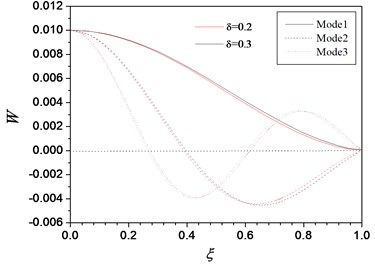Consider a FGM Mindlin circular plate with and without elastic foundation which is simply supported or free edge. Table 8 shows the effect of uniform temperature rise and heat conduction on the free vibration of FGM Mindlin circular plates with simply supported edge without elastic foundation. It can be found that for the same value of both uniform and non-uniform rises, the first-three frequency parameters of FGM Mindlin plate are decreased by increasing the power law index $p$. Subjected to the same temperature loads, the first-three frequency parameters decrease with increasing the ratio of the thickness to the radius in Table 4 and 7, but it is not always true for the FGM moderately thick circular plates with simply supported edge due to effect of heat conduction in Table 8. Also, the influence of two-parameter elastic foundation on the first-three frequencies of FGM circular plates in room and uniform temperature rise is exhibited in Table 9. As we expect, the elastic foundation has significant effects on the first-three frequencies of FGM Mindlin plates subjected to uniform temperature and heat conduction, the results are bigger than the frequencies without elastic foundation. Subjected to room temperature, uniform temperature rises and heat conduction, the second and third-order frequencies for FGM moderately thick circular plates with free edge are calculated (see Tables 10-11). It also can be found that the frequency in case of uniform heating is smaller than that of the non-uniform temperature rise. Also, one can see that increasing the thickness of the circular plate, the first-three frequency parameters decrease.

Table 8Dimensionless natural frequencies ωn of FGM thick circular plates with simply supported edge (Kw=Kg= 0)

 $\delta$ $\omega$ ${T}_{c}={T}_{m}=$ 325 K ${T}_{c}=$ 350 K, ${T}_{m}=$ 300 K $p=$ 0 $p=$ 0.2 $p=$ 0.5 $p=$ 1 $p=$ 2 $p=$ 5 $p=$ 0 $p=$ 0.2 $p=$ 0.5 $p=$ 1 $p=$ 2 $p=$ 5 0.2 1 3.6883 3.4191 3.2044 3.0511 2.9396 2.8112 3.7344 3.4556 3.2311 3.0718 3.9562 2.8257 2 24.046 22.038 20.323 18.909 17.703 16.467 24.082 22.066 20.344 18.926 17.717 16.479 3 51.437 47.174 43.468 40.312 37.526 34.705 51.478 47.206 43.492 40.331 37.542 34.719 0.25 1 4.0256 3.7072 3.4449 3.2441 3.0857 2.9158 4.0525 3.7286 3.4607 3.2564 3.0957 2.9246 2 22.613 20.728 19.105 17.746 16.568 15.368 22.638 20.747 19.119 17.758 16.578 15.377 3 46.006 42.222 38.901 36.027 33.451 30.856 56.035 42.245 38.918 36.040 33.462 30.866 0.3 4.1439 3.8063 3.5247 3.3033 3.1234 2.9345 4.1619 3.8207 3.5354 3.3116 3.1302 2.9406 21.119 19.365 17.844 16.556 15.423 14.275 21.137 19.379 17.855 16.564 15.430 14.281 41.296 37.925 34.941 32.325 29.951 27.572 41.318 37.943 34.955 32.335 29.960 27.580

Table 9Dimensionless natural frequencies ωn of FGM thick circular plates with simply supported edge (Kw= 50, Kg= 5)

 $\delta$ $\omega$ ${T}_{c}={T}_{m}=$ 325 K ${T}_{c}=$ 350 K, ${T}_{m}=$ 350 K $p=$ 0 $p=$ 0.2 $p=$ 0.5 $p=$ 1 $p=$ 2 $p=$ 5 $p=$ 0 $p=$ 0.2 $p=$ 0.5 $p=$ 1 $p=$ 2 $p=$ 5 0.2 1 9.5876 9.1729 8.7978 8.5058 8.2371 7.9820 9.4763 9.0761 8.7311 8.4373 8.1825 7.9404 2 27.798 25.792 24.051 22.663 21.450 20.238 27.601 25.618 23.929 22.534 21.344 20.155 3 55.194 50.936 47.210 44.113 41.359 38.603 54.960 50.725 47.060 43.953 41.226 38.498

Table 10Dimensionless natural frequencies ωn of FGM thick circular plates with free edge (ν=0.3, Kw=Kg= 0)

 $\delta$ $\omega$ ${T}_{c}=$ 300 K, ${T}_{m}=$ 300 K ${T}_{c}=$ 400 K, ${T}_{m}=$ 400 K $p=$ 0 $p=$ 0.2 $p=$ 0.5 $p=$ 1 $p=$ 2 $p=$ 5 $p=$ 0 $p=$ 0.2 $p=$ 0.5 $p=$ 1 $p=$ 2 $p=$ 5 0.2 2 8.0032 7.3311 6.7677 6.3221 5.9564 5.5768 7.8281 7.1795 6.6373 6.2119 5.8657 5.5042 3 30.343 27.779 25.596 23.800 22.264 20.689 30.082 27.555 25.402 23.635 22.126 20.578 0.25 2 7.9352 7.2618 6.6958 6.2437 5.8689 5.4817 7.8210 7.1629 6.6107 6.1715 5.8092 5.4335 3 28.075 25.707 23.681 21.995 20.536 19.044 27.895 25.552 23.547 21.881 20.440 18.966 0.3 2 7.7676 7.1050 6.5474 6.0994 5.7257 5.3399 7.6855 7.0340 6.4862 6.0473 5.6824 5.3048 3 25.879 23.702 21.832 20.262 18.889 17.488 25.745 23.586 21.732 20.176 18.817 17.429

Table 11Dimensionless natural frequencies ωn of FGM thick circular plates with free edge (ν= 0.3, Tc= 300 K, Tm= 400 K, Kw=Kg= 0)

 $\delta$ $\omega$ Mindlin solution Reissner solution $p=$ 0.0 $p=$ 0.2 $p=$ 0.5 $p=$ 1 $p=$ 2 $p=$ 5 $p=$ 0 $p=$ 0.2 $p=$ 0.5 $p=$ 1 $p=$ 2 $p=$ 5 0.2 2 7.8281 7.1795 6.6373 6.2119 5.8657 5.5042 7.8321 7.1831 6.6405 6.2149 5.8685 5.5069 3 30.082 27.555 25.402 23.635 22.126 20.578 30.131 27.598 25.442 23.672 22.163 20.613 0.25 2 7.8210 7.1629 6.6107 6.1715 5.8092 5.4335 7.8262 7.1675 6.6148 6.1753 5.8129 5.4370 3 27.895 25.552 23.547 21.881 20.440 18.966 27.952 25.604 23.594 21.925 20.483 19.007 0.3 2 7.6855 7.0340 6.4862 6.0473 5.6824 5.3048 7.6917 7.0395 6.4912 6.0519 5.6869 5.3092 3 25.745 23.586 21.732 20.176 18.817 17.429 25.679 23.643 21.832 20.225 18.864 17.475

Figs. 7 and 8 show the anterior three-order modes of vibration for FGM circular plates with simply supported and free edge. Also, the mode diagrams are in good agreement with the boundary conditions.

Fig. 7Anterior third-order modes of FGM moderately thick circular plate with simply supported edge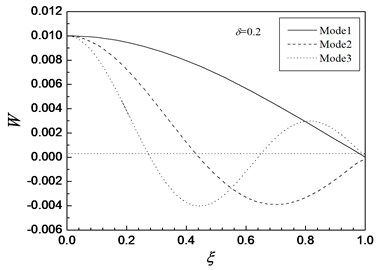Fig. 8Anterior third-order modes of the FGM moderately thick circular plate free edge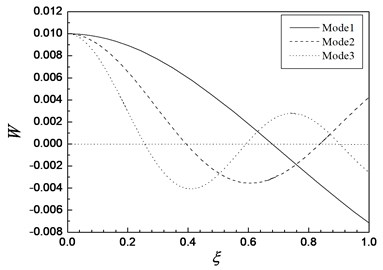## 5. Conclusions

In this paper, the free vibration of functionally graded moderately thick circular plates resting on two-parameter elastic foundations in thermal environment has been presented by employing the numerical shooting technique. Investigations on vibration of FGM thick circular plates in thermal environment with three different boundaries are also introduced. Material primary parameters are assumed temperature-dependent and vary along the thickness of the plate. By employing a shooting method, the non-linear governing equations are solved numerically and the natural frequencies of the circular plates are obtained. The effects of uniform temperature rise and heat conduction, material constant, boundary conditions and elastic foundations on the natural frequency parameters are discussed in detail. Looking into the present results, one may conclude as follows.

1) The natural frequency parameters of vibration are decreases with increasing the ratio of the thickness to the radius for both the homogeneous ceramics plate and FGM plate without thermal environment, but it is not always true for a FGM plate with simply supported edge due to effect of heat conduction. The uniform temperature rise has more effect than the non-uniform temperature rises on the frequency parameters.

2) All the first-three order frequencies of the FGM moderately thick circular plates in thermal environment decrease monotonously with the increase of the value of $p$, the reason is that the increase of the volume fraction of the metal reduces the bending stiffness of the whole plate.

3) The effect of elastic foundation on the free vibration is significant. The natural frequency of vibration for the plate is lower than the plates with elastic foundation. Also, the boundary conditions have large influence on vibration frequency of the thick plates. The natural frequency parameters are maximum with fixed boundary condition but it is minimum under free boundary condition.

4) The solutions can be used as benchmark for other numerical methods.

07 October 2016
Accepted
13 April 2017
Published
30 September 2017
SUBJECTS
Modal analysis and applications
Keywords